Скачать презентацию EQUIPMENT COSTS Peters Timmerhaus West PURCHASED

992e296b42ed4e9d1f1c01a8eddb10ef.ppt

• Количество слайдов: 15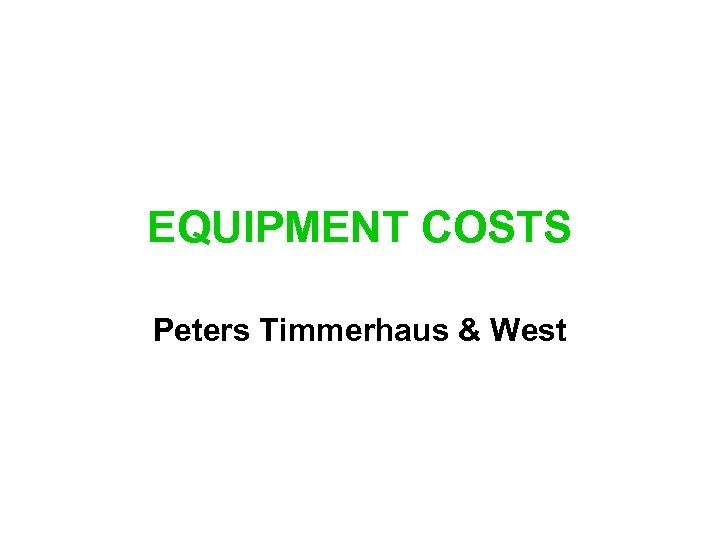EQUIPMENT COSTS Peters Timmerhaus & West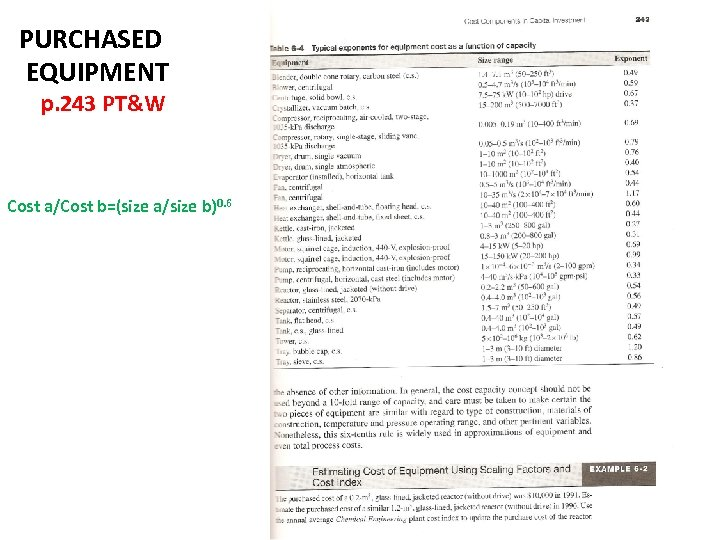PURCHASED EQUIPMENT p. 243 PT&W Cost a/Cost b=(size a/size b)0. 6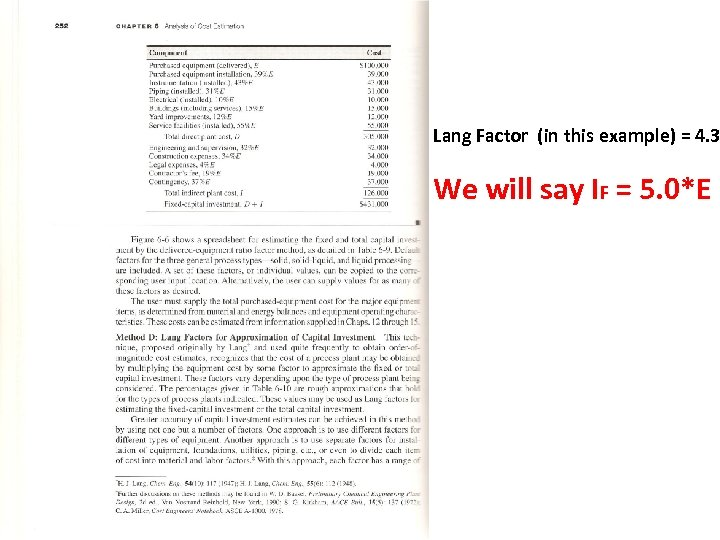Lang Factor (in this example) = 4. 3 We will say IF = 5. 0*E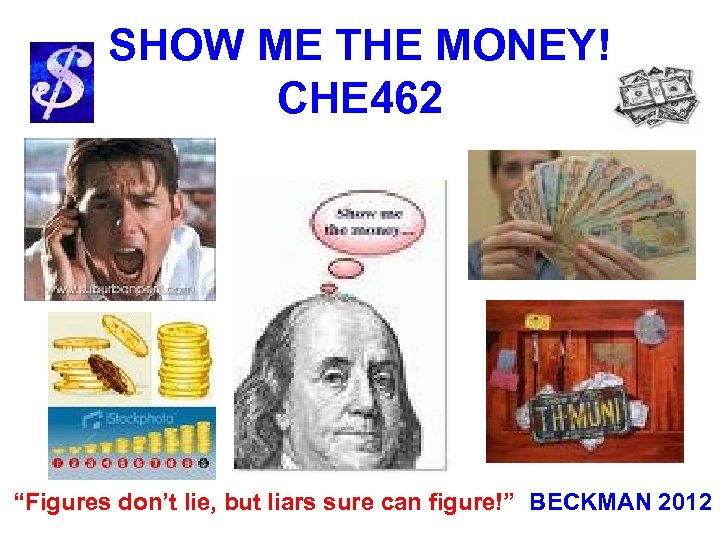SHOW ME THE MONEY! CHE 462 “Figures don’t lie, but liars sure can figure!” BECKMAN 2012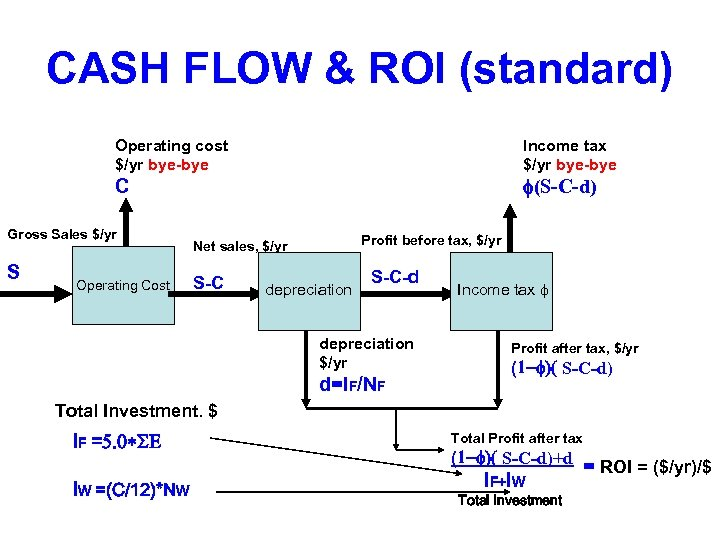CASH FLOW & ROI (standard) Operating cost \$/yr bye-bye Income tax \$/yr bye-bye f(S-C-d) C Gross Sales \$/yr S Operating Cost Profit before tax, \$/yr Net sales, \$/yr S-C depreciation S-C-d depreciation \$/yr d=IF/NF Income tax f Profit after tax, \$/yr (1 -f)( S-C-d) Total Investment. \$ IF =5. 0*SE IW =(C/12)*NW Total Profit after tax (1 -f)( S-C-d)+d IF+IW Total Investment = ROI = (\$/yr)/\$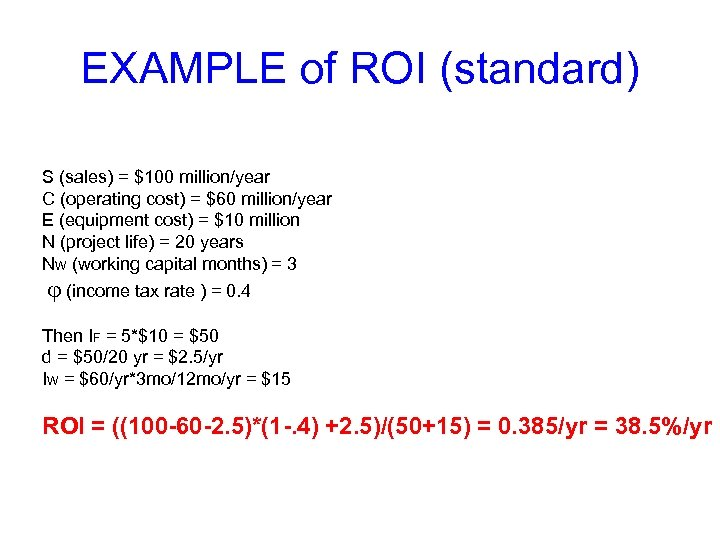EXAMPLE of ROI (standard) S (sales) = \$100 million/year C (operating cost) = \$60 million/year E (equipment cost) = \$10 million N (project life) = 20 years NW (working capital months) = 3 j (income tax rate ) = 0. 4 Then IF = 5*\$10 = \$50 d = \$50/20 yr = \$2. 5/yr IW = \$60/yr*3 mo/12 mo/yr = \$15 ROI = ((100 -60 -2. 5)*(1 -. 4) +2. 5)/(50+15) = 0. 385/yr = 38. 5%/yr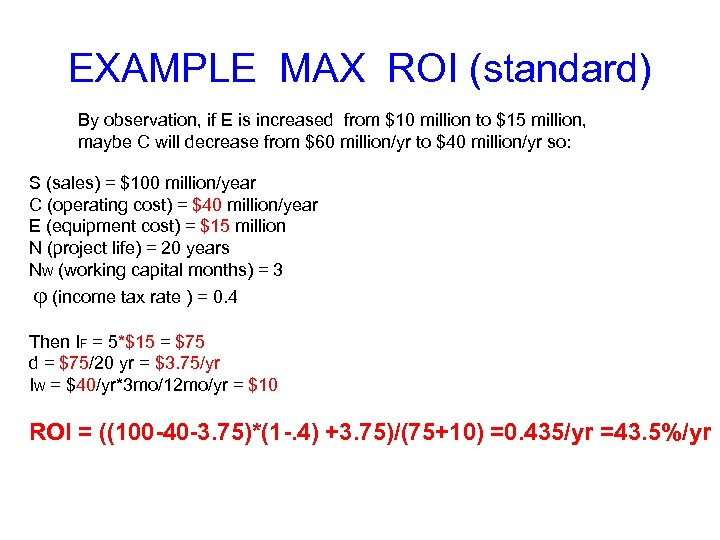EXAMPLE MAX ROI (standard) By observation, if E is increased from \$10 million to \$15 million, maybe C will decrease from \$60 million/yr to \$40 million/yr so: S (sales) = \$100 million/year C (operating cost) = \$40 million/year E (equipment cost) = \$15 million N (project life) = 20 years NW (working capital months) = 3 j (income tax rate ) = 0. 4 Then IF = 5*\$15 = \$75 d = \$75/20 yr = \$3. 75/yr IW = \$40/yr*3 mo/12 mo/yr = \$10 ROI = ((100 -40 -3. 75)*(1 -. 4) +3. 75)/(75+10) =0. 435/yr =43. 5%/yr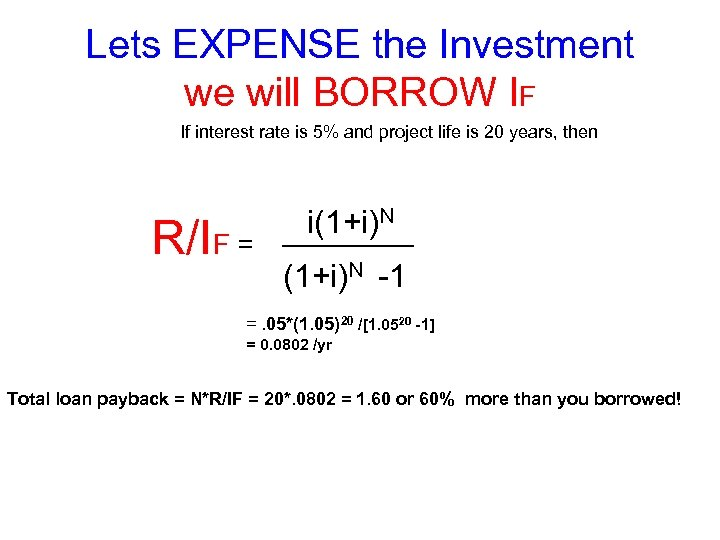Lets EXPENSE the Investment we will BORROW IF If interest rate is 5% and project life is 20 years, then R/IF = i(1+i)N -1 =. 05*(1. 05)20 /[1. 0520 -1] = 0. 0802 /yr Total loan payback = N*R/IF = 20*. 0802 = 1. 60 or 60% more than you borrowed!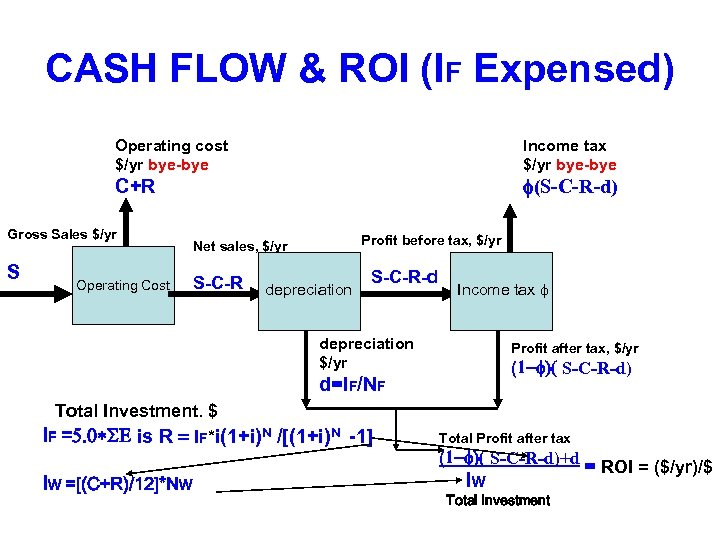CASH FLOW & ROI (IF Expensed) Operating cost \$/yr bye-bye Income tax \$/yr bye-bye f(S-C-R-d) C+R Gross Sales \$/yr S Operating Cost Profit before tax, \$/yr Net sales, \$/yr S-C-R depreciation S-C-R-d Income tax f depreciation \$/yr Profit after tax, \$/yr (1 -f)( S-C-R-d) d=IF/NF Total Investment. \$ IF =5. 0*SE is R = IF*i(1+i)N /[(1+i)N -1] IW =[(C+R)/12]*NW Total Profit after tax (1 -f)( S-C-R-d)+d IW Total Investment = ROI = (\$/yr)/\$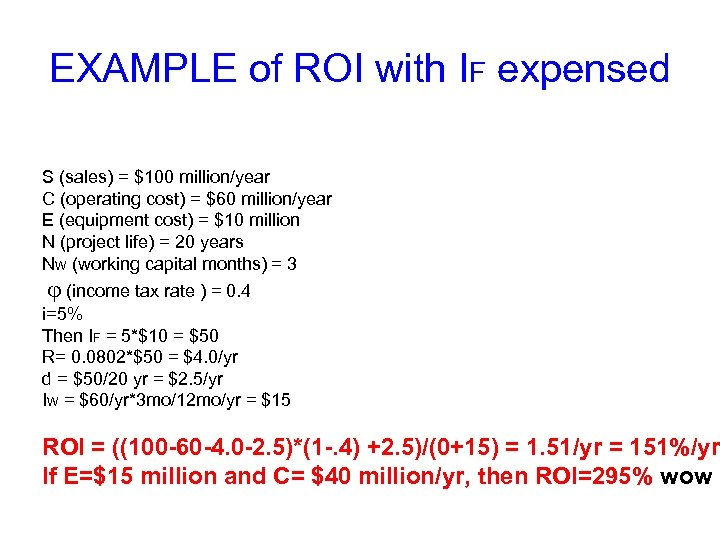EXAMPLE of ROI with IF expensed S (sales) = \$100 million/year C (operating cost) = \$60 million/year E (equipment cost) = \$10 million N (project life) = 20 years NW (working capital months) = 3 j (income tax rate ) = 0. 4 i=5% Then IF = 5*\$10 = \$50 R= 0. 0802*\$50 = \$4. 0/yr d = \$50/20 yr = \$2. 5/yr IW = \$60/yr*3 mo/12 mo/yr = \$15 ROI = ((100 -60 -4. 0 -2. 5)*(1 -. 4) +2. 5)/(0+15) = 1. 51/yr = 151%/yr If E=\$15 million and C= \$40 million/yr, then ROI=295% wow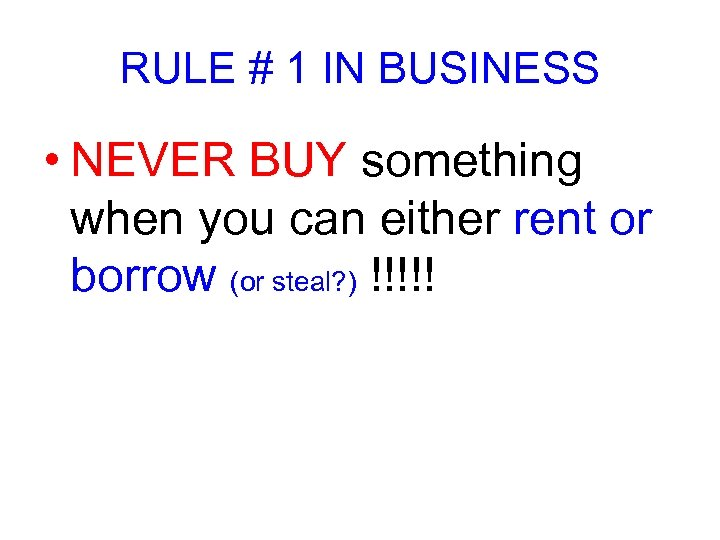RULE # 1 IN BUSINESS • NEVER BUY something when you can either rent or borrow (or steal? ) !!!!!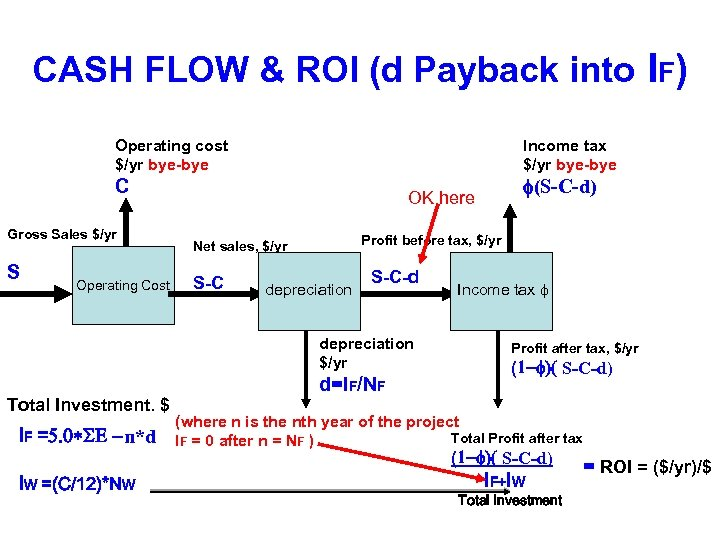CASH FLOW & ROI (d Payback into IF) Operating cost \$/yr bye-bye Income tax \$/yr bye-bye C Gross Sales \$/yr S Operating Cost Profit before tax, \$/yr Net sales, \$/yr S-C depreciation S-C-d depreciation \$/yr Total Investment. \$ IF =5. 0*SE -n*d IW =(C/12)*NW f(S-C-d) OK here d=IF/NF Income tax f Profit after tax, \$/yr (1 -f)( S-C-d) (where n is the nth year of the project Total Profit after tax IF = 0 after n = NF ) (1 -f)( S-C-d) IF+IW Total Investment = ROI = (\$/yr)/\$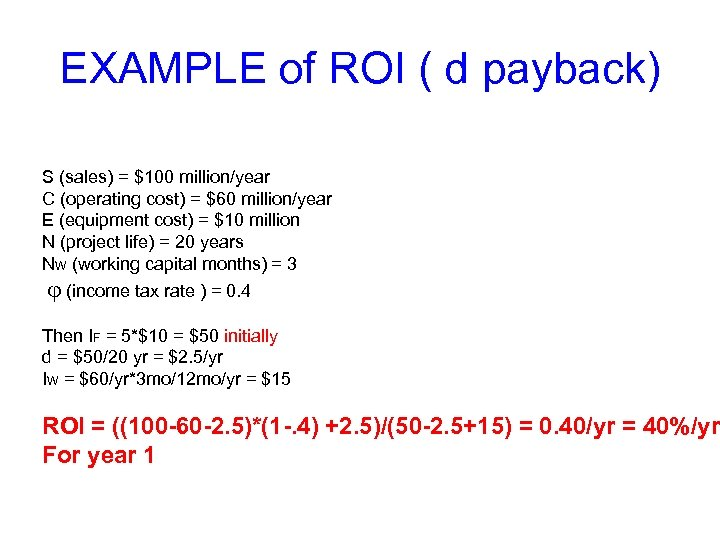EXAMPLE of ROI ( d payback) S (sales) = \$100 million/year C (operating cost) = \$60 million/year E (equipment cost) = \$10 million N (project life) = 20 years NW (working capital months) = 3 j (income tax rate ) = 0. 4 Then IF = 5*\$10 = \$50 initially d = \$50/20 yr = \$2. 5/yr IW = \$60/yr*3 mo/12 mo/yr = \$15 ROI = ((100 -60 -2. 5)*(1 -. 4) +2. 5)/(50 -2. 5+15) = 0. 40/yr = 40%/yr For year 1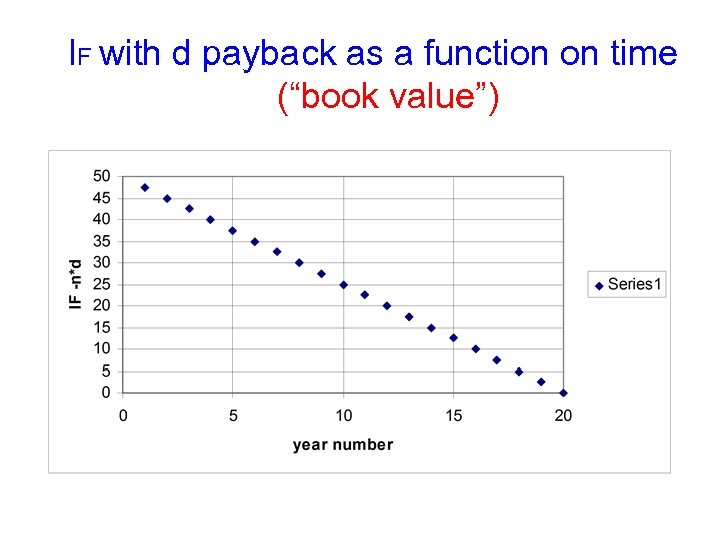IF with d payback as a function on time (“book value”)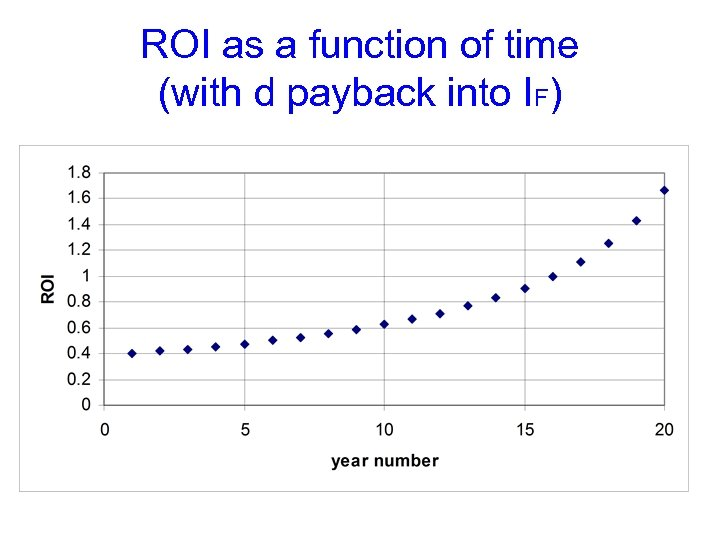ROI as a function of time (with d payback into IF)